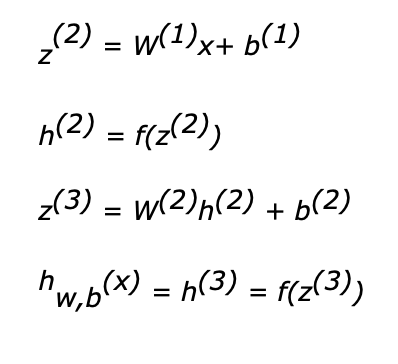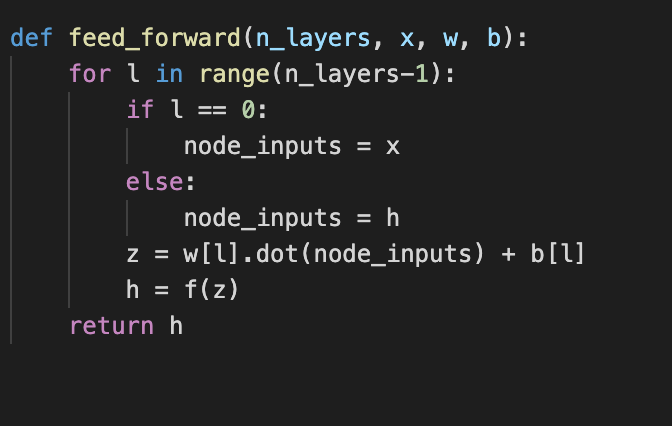•Krishna Kankipati

# Artificial Neural Networks(Part 2)- Structure of a Simple Neural Network and its implementation.

The Part 1 shows the details about an artificial node. Now let us understand a simple neural network structure and implement it using Python.

A simple neural network consists of 3 layers: an Input layer, a Hidden layer and an Output layer.A simple Neural Network Structure

We are going to use the following notations…Outputs from each node in Feed-forward propagationthe function f(), refers to the activation function(here we are using sigmoid function). From Layer 2 h1, h2, h3 are output variables which act as inputs to the node in Layer 3. Details about the sigmoid function can be referred from Part 1.

Implementing a feed-forward function…In the above program we have used ‘for’ loops for calculations. Well, for small number of input values are suited, what if there are more than 10 input values i.e 10 input nodes, and more than 2 hidden layers?

Vectorisation

Vectorisation is nothing but a dot product between two numerical matrices. In the above code, we used for loops to multiply weights and the input values. This can be modified by performing a dot product between the weight matrix and the input matrix.Thus the above equations can be replaced as the followingThe reduced equation for the three layered network. W represent the weights matrix, z represent the summated input vector, b represents the bias weights and h represents the output of a node. Thus we can generalize the whole equations into a simpler form for better understanding.A feed-forward equation

Understanding the Vectorisation by observing the matrix multiplication.Thus the above code can be simplified asIn Part 3 we’ll discuss about Loss and Cost functions, and Gradient Descent.

See All